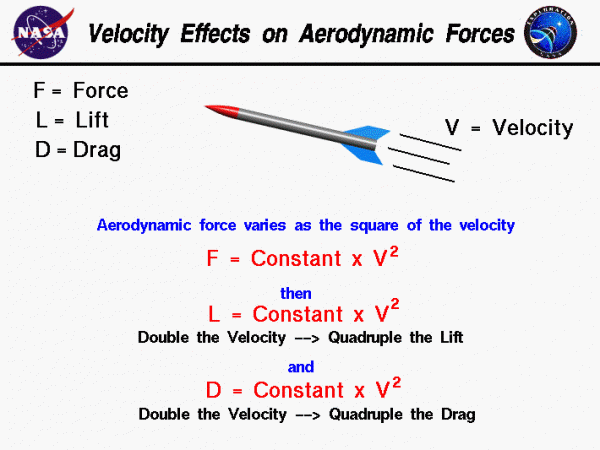# Velocity Effects## Aerodynamic Forces

Aerodynamic forces are generated whenever an object moves through a liquid or gas. From Newton’s second law of motion, the aerodynamic forces on the body are directly related to the change in momentum of the fluid with time. The fluid momentum is equal to the mass times the velocity of the fluid. Since the fluid is moving, defining the mass gets a little tricky. If the mass of fluid were brought to a halt, it would occupy some volume in space; and we could define its density to be the mass divided by the volume. With a little math we can show that the aerodynamic forces are directly proportional to the density of the fluid that flows by the rocket. As a result of this derivation, we also find that lift and drag depend on the square of the velocity.

Here is the derivation, beginning with Newton’s second law:

$$\LARGE F=\frac{\text{d}(mV)}{\text{d}t}$$

where F is the force, m is the mass, t is time, and V is the velocity. If we integrate this equation, we obtain:

$$\LARGE F=\text{constant}\times V\frac{m}{t}$$

Since the fluid is moving, we must determine the mass in terms of the mass flow rate. The mass flow rate is the amount of mass passing a given point during some time interval and its units are mass/time. We can relate the mass flow rate to the density mathematically. The mass flow rate mdot ($$\dot{m}$$) is equal to the density rho ($$\bf\rho$$) times the velocity times the area A through which the mass passes.

$$\LARGE \dot{m}=\frac{m}{t}=\rho VA$$

With knowledge of the mass flow rate, we can express the aerodynamic force as equal to the mass flow rate times the velocity.

$$\LARGE F=\text{constant}\times V\rho VA$$

A quick units check:

$$\LARGE \text{mass}\times\frac{\text{length}}{\text{time}^{2}}=\text{constant}\times\frac{\text{length}}{\text{time}}\times\frac{\text{mass}}{\text{length}^{3}}\times\frac{\text{length}}{\text{time}}\times\text{length}^{2}$$

$$\LARGE \text{mass}\times\frac{\text{length}}{\text{time}^{2}}=\text{mass}\times\frac{\text{length}}{\text{time}^{2}}$$

Combining the velocity dependence and absorbing the area into the constant, we find:

$$\LARGE F=\text{constant}\times \rho V^{2}$$

The aerodynamic force equals a constant times the density times the velocity squared. The dynamic pressure of a moving flow is equal to one half of the density times the velocity squared. Therefore, the aerodynamic force is directly proportional to the dynamic pressure of the flow.

## Lift and Drag

The velocity used in the lift and drag equations is the relative velocity between an object and the flow. Since the aerodynamic force depends on the square of the velocity, doubling the velocity will quadruple the lift and drag.

You can investigate the effect of velocity and the other factors on the flight of a rocket by using the RocketModeler Java Applet.

Provide feedback